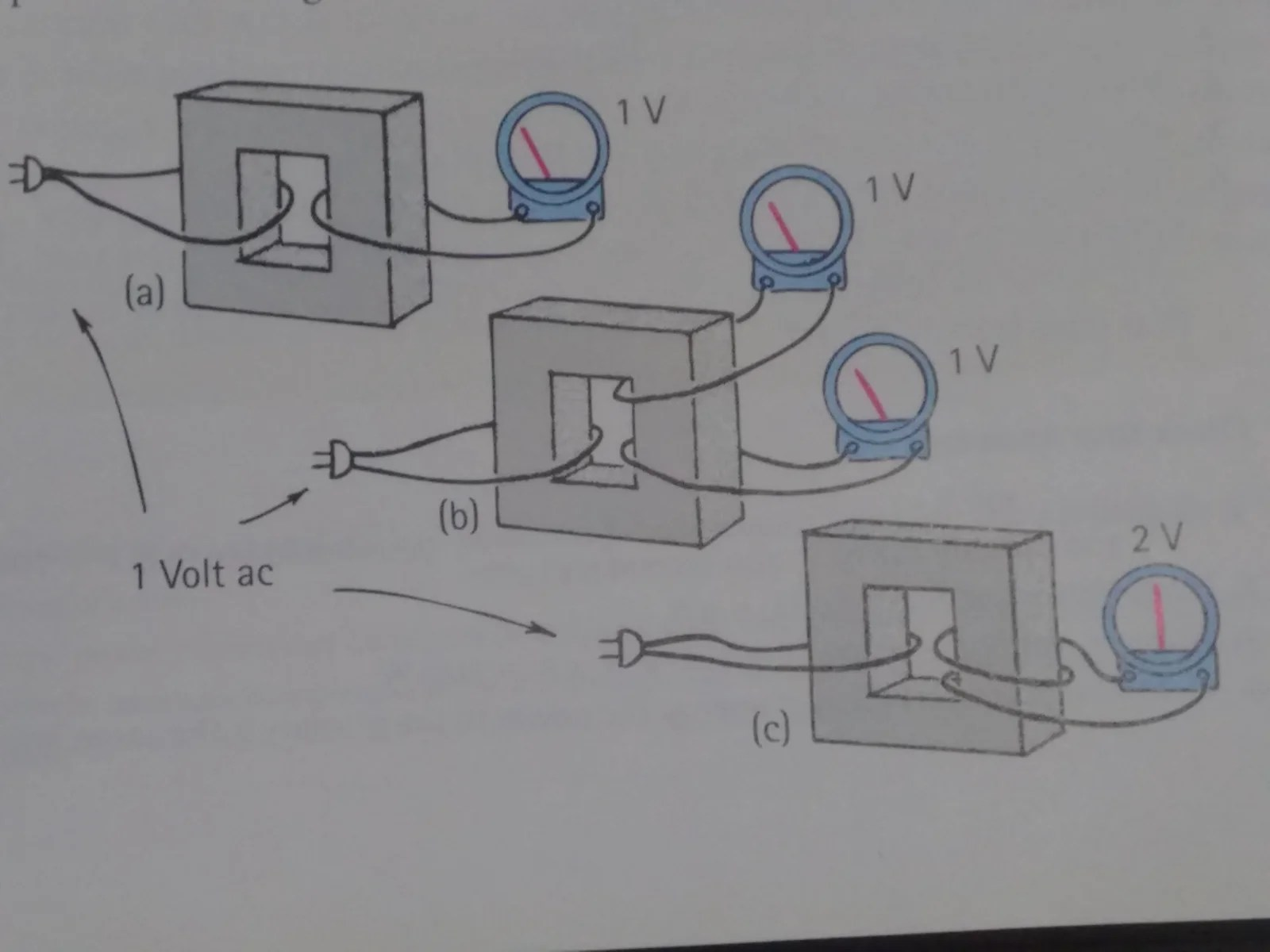# Electromagnetic Induction

#### Exam Tip

• It is important to know that the transformer changes the voltage and the current, but not the frequency whether it is an ideal or non-ideal transformer.
• In an ideal transformer, the power in secondary is equal to power in primary.
• If d.c circuit is used in primary, there will be a momentarily induced emf in the secondary coil and after that there will be no induced emf anymore. Thus, transformer cannot be used with d.c supply. In other words, direct voltage fed into the primary coil would result in zero voltage in the secondary except for the short interval of time it takes the current in the primary coil to reach its final steady value.
• The iron core in the transformer is used to ensure that as much of the magnetic flux produced in the primary coil as possible enters the secondary coil. Iron has the property that it confines magnetic flux and so magnetic field lines do not spread out into the region outside the core.
• An intuitive picture  of magnetic flux is the number of magnetic field lines that cross or pierce the loop area. If the loop has N turns of wire around it, in this case we speak of magnetic flux linkage.
• A changing magnetic flux creates an induced emf, not necessarily a current. There will be a current only if the loop is conducting, i.e if the resistance of the circuit is not infinite. For example, a loop containing an ideal voltmeter cannot let current through, but there will be an emf if the flux is changing.

#### Sources of Power Loss in Transformer

• Eddy currents: they are tiny currents created in the core because the free electrons of the core move in the presence of a magnetic field. These currents heat up the core, dissipating energy. Solution: To have a laminated core rather than a single block so to reduce (because eddy currents is just one of the factors) the power losses by eliminating the eddy currents.
• Heating of the coils themselves will result in power loss also.
• Magnetic hysteresis is a complex phenomenon. Hysteresis is derived from Greek word that means “to lag”. Due to magnetic hysteresis, the magnetic energy stored in the magnetic field as the magnitude of the field increases is not all given back as the field magnitude decreases, resulting in power loss. To put it simply, it is a phenomenon of magnetisation lagging behind the field producing it and the need for coercive force to remove the residual magnetism when the magnetic flux density of the source has already reached zero. Remedy? Use soft magnetic material with low hysteresis such as silicon steel, steel alloys and Mn-Zn ferrite, for instance.

#### Power transmission in transformers

• Cables have resistance and thus there is power loss.
• To minimise the power loss due to resistance of the cable, it is necessary to reduce the current (there is not much that can be done about reducing cable resistance). Thus, power companies supply electricity at large voltages.
• We use P =RI^2 to calculate power loss rather than P = (V^2)/R simply because in order to calculate the power loss using P =V^2/R,  V here is the voltage drop across the transmission line and not the voltage that it is being transmitted.

#### Splitting of voltage at secondary with more than one loop## How to convert ac current into a dc current?

Taken from http://www.circuitstoday.com/full-wave-bridge-rectifier

### Full Wave Rectifier Working & Operation

The working & operation of a full wave bridge rectifier is pretty simple.  The circuit diagrams and wave forms we have given below will help you understand the operation of a bridge rectifier perfectly.  In the circuit diagram, 4 diodes are arranged in the form of a bridge. The transformer secondary is connected to two diametrically opposite points of the bridge at points A & C.  The load resistance RL  is connected to bridge through points B and D.Full Wave Bridge Rectifier – Circuit Diagram with Input and Output Wave Forms

#### During the first half cycle

During first half cycle of the input voltage, the upper end of the transformer secondary winding is positive with respect to the lower end. Thus during the first half cycle diodes D1 and D3 are forward biased and current flows through arm AB, enters the load resistance RL, and returns back flowing through arm DC. During this half of each input cycle, the diodes D2 and Dare reverse biased and current is not allowed to flow in arms AD and BC. The flow of current is indicated by solid arrows in the figure above. We have developed another diagram below to help you understand the current flow quickly. See the diagram below – the green arrows indicate beginning of current flow from source (transformer secondary) to the load resistance. The red arrows indicate return path of current from load resistance to the source, thus completing the circuit.Flow of current in Bridge Rectifier

#### During the second half cycle

During second half cycle of the input voltage, the lower end of the transformer secondary winding is positive with respect to the upper end. Thus diodes D2 and D4 become forward biased and current flows through arm CB, enters the load  resistance RL,  and returns back to the source flowing through arm DA. Flow of current has been shown by dotted arrows in the figure. Thus the direction of flow of current through the load resistance RL remains the same during both half cycles of the input supply voltage.  See the diagram below – the green arrows indicate beginning of current flow from source (transformer secondary) to the load resistance. The red arrows indicate return path of current from load resistance to the source, thus completing the circuit.Path of current in 2nd Half Cycle